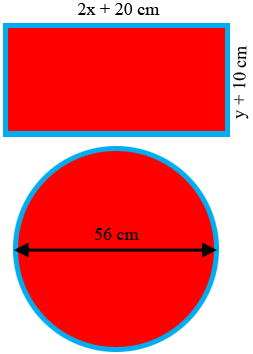SEARCH HOMEMath Central Quandaries & QueriesQuestion from Ramzan a student: a diagram shows the shape of rectangular framework with length (2x+20)cm and with (y+10)cm . The outline is made of wire . If a circle with diameter 56 cm is to be made from the wire and the area of the rectangle is 420 square centimeter ,find the possible lengths and width of the rectangleHi,The area of a rectangle is the length times the width. What is the area of the rectangle above in terms of $x$ and $y?$ But this area is 420 square centimeters so this gives you an equation in $x$ and $y.$

Since the figures are made from the same wire their perimeters are the same. This gives you a second equation in $x$ and $y.$

Solve the pair of equations for $x$ and $y.$

PennyMath Central is supported by the University of Regina and The Pacific Institute for the Mathematical Sciences.